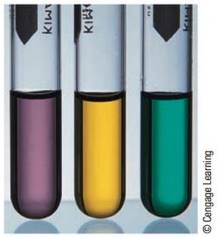# The following test tubes each contain a different chromium complex ion. For each complex ion, predict the predominant color of light absorbed. If the complex ions are Cr(NH 3 ) 6 3+ , Cr(H 2 O) 6 3+ , and Cr(H 2 O) 4 Cl 2 + , what is the identity of the complex ion in each test tube? ( Hint: Reference the spectrochemical series.)### Chemistry: An Atoms First Approach

2nd Edition
Steven S. Zumdahl + 1 other
Publisher: Cengage Learning
ISBN: 9781305079243

#### Solutions

Chapter
Section### Chemistry: An Atoms First Approach

2nd Edition
Steven S. Zumdahl + 1 other
Publisher: Cengage Learning
ISBN: 9781305079243
Chapter 20, Problem 59E
Textbook Problem
10 views

## The following test tubes each contain a different chromium complex ion.For each complex ion, predict the predominant color of light absorbed. If the complex ions are Cr(NH3)63+, Cr(H2O)63+, and Cr(H2O)4Cl2+, what is the identity of the complex ion in each test tube? (Hint: Reference the spectrochemical series.)

Interpretation Introduction

Interpretation: Three test tubes containing different complex ions are given. The color of light absorbed by each given ion is to be predicted. The identity of complex ion in each test tube is to be predicted.

Concept introduction: The capacity to absorb light by complex ion is directly proportional to crystal field splitting energy and energy absorbed is inversely proportional to the wavelength.

To determine: The color of light absorbed by each ion Cr(H2O)63+,Cr(NH3)63,Cr(H2O)4Cl2+ and the identity of complex ion in each test tube.

The order of increasing crystal field splitting energy for complex ions Cr(H2O)63+,Cr(NH3)63,Cr(H2O)4Cl2+ is,

Cr(NH3)63>Cr(H2O)63+>Cr(H2O)4Cl2+

### Explanation of Solution

Explanation

The spectrochemical series (crystal field splitting energy) of strong to weak ligand is,

CN>NO2>en>NH3>H2O>OH>F>Cl>SCN>Br

Hence, order of increasing crystal field splitting energy for complex ions Cr(H2O)63+,Cr(NH3)63,Cr(H2O)4Cl2+ is,

Cr(NH3)63>Cr(H2O)63+>Cr(H2O)4Cl2+

The energy absorbed by light is given by the expression,

E=hcλ

Where,

• E is the energy absorbed.
• h is the Plank’s constant.
• c is the velocity of light.

It is clear from the above equation that, energy absorbed is inversely proportional to the wavelength. It means that higher the energy, lower will be the wavelength.

Since, order of increasing crystal field splitting energy for complex ions Cr(H2O)63+,Cr(NH3)63,Cr(H2O)4Cl2+ is,

Cr(NH3)63>Cr(H2O)63+>Cr(H2O)4Cl2+

### Still sussing out bartleby?

Check out a sample textbook solution.

See a sample solution

#### The Solution to Your Study Problems

Bartleby provides explanations to thousands of textbook problems written by our experts, many with advanced degrees!

Get Started

Find more solutions based on key concepts
Name the following compounds. a Cu(NO2)3 b (NH4)3P c K2SO3 d Hg3N2

General Chemistry - Standalone book (MindTap Course List)

Which of the following values is found on food labels? Recommended Dietary Allowances Dietary Reference Intakes...

Nutrition: Concepts and Controversies - Standalone book (MindTap Course List)

What is population genetics?

Human Heredity: Principles and Issues (MindTap Course List)

What is an autotroph? Are all plankton autotrophic?

Oceanography: An Invitation To Marine Science, Loose-leaf Versin

A transverse traveling wave on a taut wire has an amplitude of 0.200 mm and a frequency of 500 Hz. It travels w...

Physics for Scientists and Engineers, Technology Update (No access codes included)

How can collisions affect the shapes of galaxies?

Foundations of Astronomy (MindTap Course List)